# Difference Between call, apply and bind method in JavaScript

Hi, Let’s discuss the most asked question in the interviews…
First thing call & apply are very similar there is only one difference between them which is the way we pass the arguments.

Call & apply Use to invoke a function directly by passing in the reference which points to the `this`variable in the method.
Bind creates a new function with a given
`this` value, and returns that function without executing it.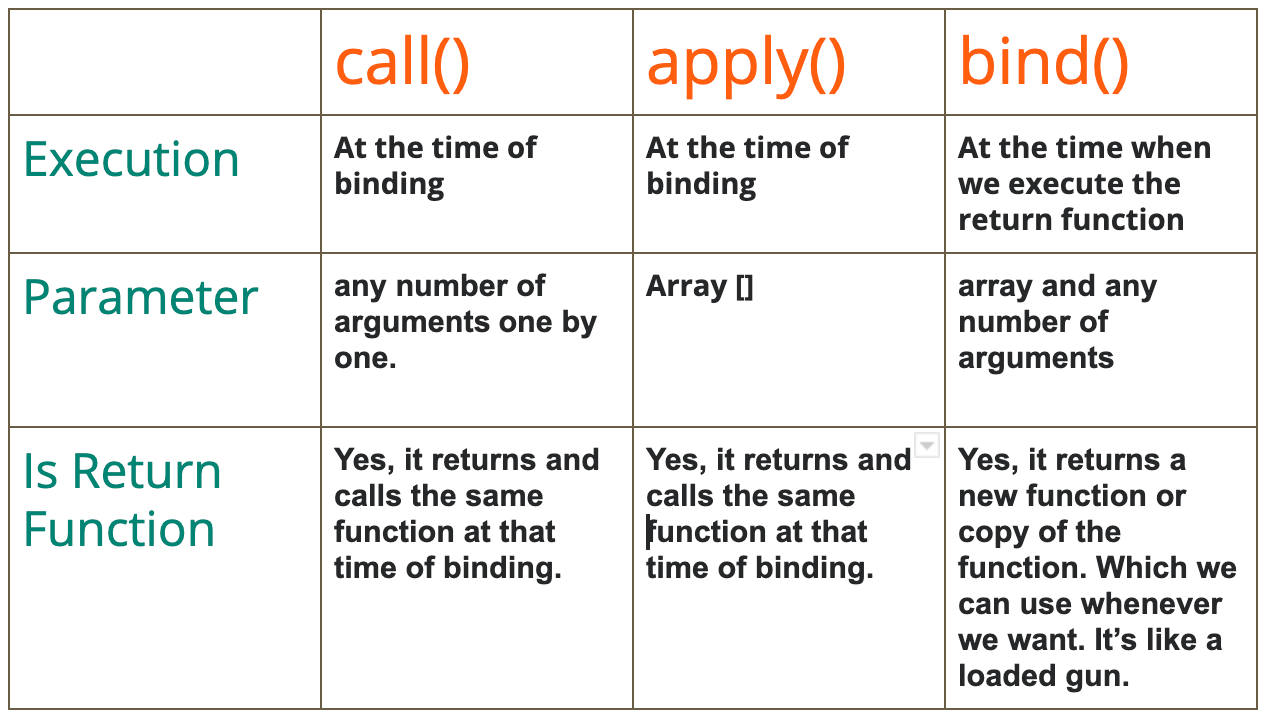Differences Between call(),apply()and bind().

Let’s discuss this by code so we will create an object with some properties and function.

And if we want to call the function we can simply call this by an object.funciton().

Let’s create one more object of the same type.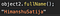Declaring & calling a function in an object

Let’s take a condition where we have 1000’s of this type of object so, in that case, we are wasting a lot of memory and time. So to overcome this situation we have a concept of call(), apply() and bind().

Let’s see how we can do this by using call().

First, we will change the object a little so the new objects will look like this. We also added some arguments in the function you can also use this without arguments.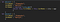Created two objects with function and one without function.

So now we want to call the fullName function for object2 so we will pass the reference of the object to the function by using the call method.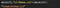with arguments

We can also use apply for the same but the only difference is the way of passing arguments. we only need to send in an array.calling apply with parameters in the array

Let’s talk about bind() bind return a method (we can say that as a callback method) and we need to call that method for executing the function.
Let see this by code.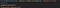Calling bind method and storing the return value in bindReturnfunction variable

This is the function return by the bind method. We need to call that function to execute our method.Return function by bind method.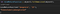Call the return function with arguments.

We can also pass the argument directly to bind or in the return function.

We can also take the function out of the object 1.

let object1 = {
firstName : “Himanshu”,
lastName : “Satija”,

}
let fullName = function (){
return this.firstName + this.lastName + city + age
}
let object2 = {
firstName : “Himanshu”,
lastName : “Satija”,
}
now calling will be
fullName.call(object1);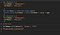calling call with a function declaring outside the object

These built-in methods, that exist on every JS function can be very useful. Even if you do not end up using them in your day to day programming, you will still run into it often when reading other peoples code

If you have any questions, as always, reach out via Linkedin

Javascript enthusiast | Frontend Developer at Anatta Design

Javascript enthusiast | Frontend Developer at Anatta Design